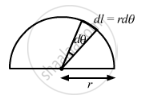Advertisement Remove all ads

# The Moment of Inertia of a Uniform Semicircular Wire of Mass M and Radius R About a Line Perpendicular to the Plane of the Wire Through the Centre is - Physics

MCQ
Fill in the Blanks

The moment of inertia of a uniform semicircular wire of mass M and radius r about a line perpendicular to the plane of the wire through the centre is ___________ .

#### Options

• $M r^2$

• $\frac{1}{2}M r^2$

• $\frac{1}{4}M r^2$

• $\frac{2}{5}M r^2$

Advertisement Remove all ads

#### Solution

$M r^2$

Consider an element of length, dl = rdθ.

$dm = \frac{M}{\pi r}dl = \frac{M}{\pi r}rd\theta$

MOI of semicircular wire = $\int_0^\pi r^2 dm$

$I = \int_0^\pi r^2 \frac{m}{\pi r}rd\theta$

$\Rightarrow I = m r^2$Is there an error in this question or solution?
Advertisement Remove all ads

#### APPEARS IN

HC Verma Class 11, 12 Concepts of Physics 1
Chapter 10 Rotational Mechanics
MCQ | Q 14 | Page 193
Advertisement Remove all ads

#### Video TutorialsVIEW ALL 

Advertisement Remove all ads
Share
Notifications

View all notifications

Forgot password?